# Magnetic Effects of Electric Current-Class 10

CONTENT LIST

## Magnetic Effects of Electric Current

A relationship between electricity and magnetism had been discovered in 1820, by Hans Christian Oersted .

When we brought a compas near a current carrying conductor then the needle of this compass gets deflected . This is due to magnetic effects of current.

When an electric current is passed through a wire, it behaves like a magnet. This effect of current is  called the magnetic effect of the electric current.

The relation between electricity and current is called electromagnetism.

### MAGNETIC FIELD AND FIELD LINES

Magnetic Field:
When a bar magnet is  freely suspended from its centre by a thread, one end of magnet points towards north direction  of  the Earth called  North pole and other end of magnet points toward the South of earth called south pole.

The  region or space around a magnet within which it's  influence can be realized by another magnet is called magnetic field.

Field lines for a bar magnet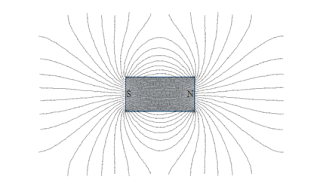Properties of magnetic fields of lines

Magnetic lines of force are imaginary  lines around a  magnet, which  shows the direction in which a magnetic material will be aligned when placed at the location.

Magnetic field lines are always parallel to each other in the uniform magnetic field. Magnetic field lines do not cross each other.

Each Magnetic field lines always forms a closed loops.

Magnetic field lines are directed from the North Pole to the South Pole of the magnet in outside region of magenet, while inside the magnet they are directed from the South Pole to North Pole of the magnet.

The relative strength of magnetic field is shown by the degree of closeness of the field lines. Field lines are more crowded where the field is stronger.### MAGNETIC FIELD DUE TO A CURRENT-CARRYING ARRYING CONDUCTOR

Moving charges produce magnetic fields which are proportional to the current and hence a current carrying conductor creates magnetic effect around it.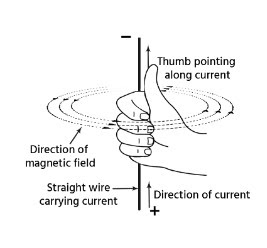#### Magnetic Field due to a Current through a Straight Conductor

Magnetic field  produced due to a current-carrying conductor depends on the magnitude  and direction of  current in the conductor and distance of the point (where magnetic field is calculated ) from the conductor.

The direction of the magnetic field  produced in current carrying  wire is perpendicular to the wire.

The SI unit of the magnetic field is Tesla and denoted by B.

#### Characteristics Of Magnetic Field Due To Current Carrying Conductor

The magnetic field developed due to a current-carrying conductor has some characteristics:

1. Magnetic field  lines encircle the conductor.
2. Magnetic field  lines lies in a plane perpendicular to the conductor.
3. Reversal in direction of current flow in wire reverses the direction of the field.
4. Magnetic field's strength is directly proportional to the magnitude of current.
5. Magnetic field strength at any point  around the current  carrying  conductor is inversely proportional to the distance of the point from the wire.

#### Right-Hand Thumb Rule

If you curl your right hand’s fingers around the current carrying wire with your thumb pointing towards direction of the current, then the direction in which the fingers would curl will give the direction of the magnetic field.

#### Magnetic Field due to a Current through a Circular Loop

If this straight wire is bent in the form of a circular loop, and a current is passed through it then every point of a current-carrying circular loop creates  the concentric circular magnetic field lines around circular wire throughout  the periphery and that would become larger and larger as we move away from the wire .

At the centre of the circular loop, the arcs of thes magnetic  field  lines make big circles ,that would appear as straight lines.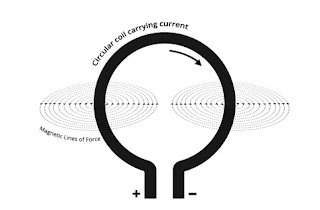#### Magnetic Field due to a Current in a Solenoid

Coil of many circular turns of insulated copper wire wrapped closely in the shape of a cylinder is called a solenoid.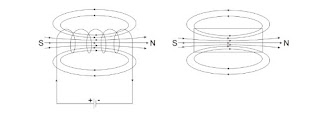Field strength created by a current-carrying wire at a particular point depends directly on the current passing through it. Therefore, in a circular coil ,that has 'n' turns in coil, the field created is 'n' times as large as that point (p) created by a single turn & the field strength due to each turn adds up at the centre of coil.

One end of the solenoid acts as a north pole, while the other behaves as the south pole.

The field lines inside the solenoid are in the form of parallel straight lines. This indicates that the magnetic field is the same at all points inside the solenoid

A strong magnetic field produced inside a solenoid can be used to magnetise a piece of magnetic material, like soft iron, when placed inside the coil  The magnet so formed is called an electromagnet.

### FORCE ON A CURRENT-CARRYING CONDUCTOR

When a current carrying conductor is kept in a magnetic field. A force is the  experienced on the conductor and in a direction perpendicular to both the direction of magnetic field and the direction of current  in the conductor.

The direction of force depend on the direction of current in conductor

This force is also known as Lorentz force.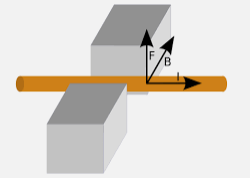The direction of this force can be determined  either by Fleming’s left hand rule or by right hand palm rule.

### ELECTRIC MOTOR

An electric motor is used to converts the electrical energy into the mechanical energy.

Principle: An electric motor works on the principle that when an electric current is passed through a conductor kept perpendicular to the field, a force acts on the conductor and  as a result of which the conductor begins to move and  gives out mechanical energy .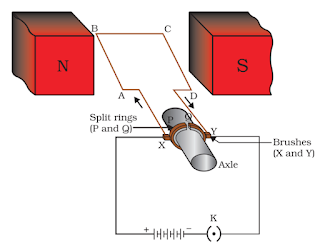It is made of a rectangular coil ABCD of insulated copper wire. The coil mounted on shaft is placed between the two poles of a magnetic field such that the arm AB and CD are perpendicular to the direction of the magnetic field.

The ends part of the coil are connected to the two halves P and Q of a split ring.The inner sides of these halves are insulated and attached to an axle.

The external conducting edges of halves P and Q touch two conducting stationary brushes X and Y.

Current enters in the coil ABCD from the source battery through conducting brush X and flows back to the battery through brush Y.

The current in arm AB of the coil flows in direction from A to B. In arm CD ,current flows from C to D, Which is, opposite to the direction of current through arm AB.

The force acting on arm AB pushes it downwards while the force acting on arm CD pushes it upwards.

The direction of current reveresd in the coil with the help of split ring also called commutator and force acting on AB and CD gets reveresd ,which gives the turing motion in shaft .

Thus the coil and the axle O, Which is mounted free to turn about an axis, rotate anti-clockwise.

### ELECTROMAGNETIC INDUCTION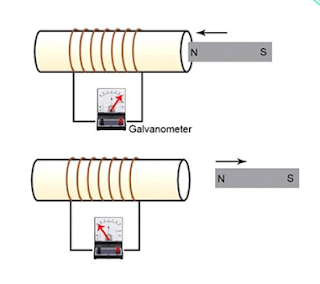Conduct an experiment ,connect ends of coil through a galvenometer ( an instrument used to detect and measure small amounts of current in an electrical circuit).

Bring a bar magenet towards the coil sharply, galvenomter will get deflected in a particular direction.This show current in coil .When the magnet come to rest, there is no deflection in galvenometer.which means no cuurent in coil .

Now reveres the experiment, move the magnet away from the coil ,again we observes the deflection galvenometer niddle in oppsite direction. Which shows current in coil in oppsite direction

This experiment shows change in magenetic filed aroound a conducting wire genertes potetial diffrence in conductor .This potentila diffrence is called electromotive force (emf).

Electromagnetic induction is a process of creation of an electro-motive force (EMF) by way of a changing magnetic field around an electric conductor.

Electromagnetic induction was discovered by Michel Fradey in 1831

Right hand palm rule

Right hand palm rule states if we stretch our right hand such that thumb and rest of the fingers are mutually perpendicular , and fingers show the direction of magnetic field lines , thumb shows the direction of current then a perpendicular on the palm will show the direction of force on current carrying wire.

### ELECTRIC GENARATOR

An electric generator is an electrical device that converts the mechanical energy into electrical energy.

The electric generators are operated on principles of electromagnetic induction.

In Electric genarator , a straight conductor moves in a magnetic field and then the current is produced in the conductor.

A conducting coil tightly wound onto a metal core is rotated rapidly between the poles of a horseshoe type magnet.

The conducting coil along with its core is known as an armature.

The armature is connected to a shaft of a mechanical energy source such as a motor and which rotates the armeture in magenetic filed.

When the coil rotates in side the poles of magenet, it cuts the magnetic field which lies between the two poles of the magnet.

Thus an electric current produce in the coil due to electromagnetic induction.

### DOMESTIC ELECTRIC CIRCUITS

Electricity is produced at the power station (2kV-25kV) .

Volatge of current is increased at power station out srouce by means of step up transfomer (400kV) and tranmited through national grid.

Further voltage of national grid current reduced by means of step down transfommer to 25 kV/11kV at substation.

Again voltage reduced at power house to 220-230 V and supped to the house.

Two wires brought to home from power house wire network , one is Line/main(L) and another is Neutral(N). thus potential difference between Lines and Neutral is (230-0=230V).

Question: Name the device is used to produce electricity.
Question: Which of below express the meaning of electromagnetic induction?
(A) Charging of an electric conductor.
(B) Production of magnetic field due to a current flowing through a coil.
(C) Generation of a current in a coil due to relative motion between the coil and the magnet.
(D) Motion of the coil around the axle in an electric motor.
Answer: (C) Generation of a current in a coil due to relative motion between the coil and the magnet.
Question: Whats happen during short circuit?
(A) the current in the circuit reduces substantially.
(B) the current in the circuit does not change.
(C) the current in the circuit increases heavily.
(D) the current in the circuit vary continuously.
Answer: (C) the current in the circuit increases heavily.
Question: What is the frequency of AC (alternating current) in India .
(A) 50 Hz
(B) 60 Hz
(C) 110 Hz
(D) 220 Hz
Question: Which of the following statement correctly describes the magnetic field near a long straight wire carrying current?
(A) The field consists of straight lines perpendicular to the wire.
(B) The field consists of straight lines parallel to the wire.
(C) The field consists of radial lines originating from the wire.
(D) The field consists of concentric circles centred on the wire.
Answer: (D) The field consists of concentric circles centred on the wire.
Question: When electric current passes through a wire , it behaves like a magnet. This effect of cuurent is known as ________.
(A) Magnetic effect of current
(B) Electrical effect of current
(C) Heating effect of current
(D) Optical effect of current
Question: Who discovered that when a compass needle deflects when kept nearer to an electric circuit in which current is flowing?
(A) Hans Christian Oersted
(C) Galileo (D)Newton
Question: The magnetic field produced due to a current through a circular loop depends directly on which of below option.
(B) current passing through it
(C) resistance of the wire
(D) thickness of the wire
Question: The direction of the magnetic field around a straight conductor carrying current is given by ________.
(A) Right hand thumb rule
(B) Fleming’s left hand rule
(C) Fleming’s right hand rule
(D) None of these
Question: Which of following describe the magnetic field lines outside a bar magnet:
(A) Originate from the South pole and end at its North Pole
(B) Originate from the North pole and end at its East Pole
(C) Originate from the North Pole and end at its South Pole
(D) Originate from the South pole and end at its West Pole
Answer:(C) Originate from the North Pole and end at its South Pole
Question: A soft iron bar is placed inside a current-carrying solenoid. The magnetic field inside the solenoid:
(A) Will decrease
(B) Will increase
(C) Will become zero
(D) Will remain the same